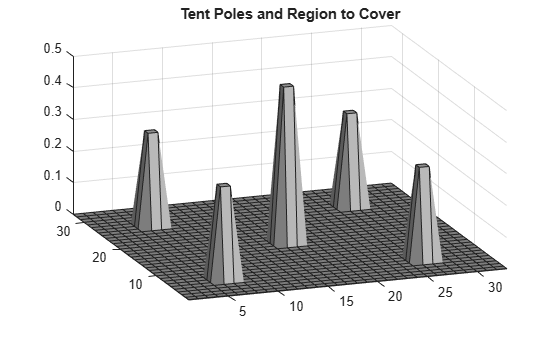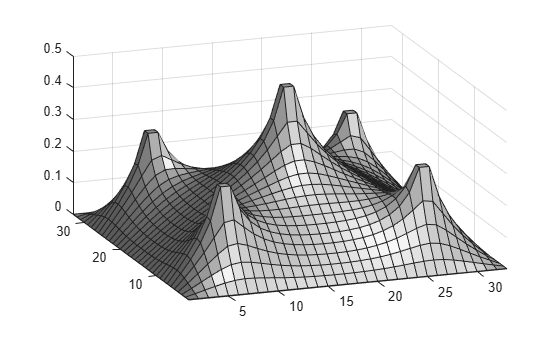This example shows how to determine the shape of a circus tent by solving a quadratic optimization problem. The tent is formed from heavy, elastic material, and settles into a shape that has minimum potential energy subject to constraints. A discretization of the problem leads to a bound-constrained quadratic programming problem.

For a solver-based version of this example, see Bound-Constrained Quadratic Programming, Solver-Based.

### Problem Definition

Consider building a circus tent to cover a square lot. The tent has five poles covered with a heavy, elastic material. The problem is to find the natural shape of the tent. Model the shape as the height x(p) of the tent at position p.

The potential energy of heavy material lifted to height x is cx, for a constant c that is proportional to the weight of the material. For this problem, choose c = 1/3000.

The elastic potential energy of a piece of the material ${E}_{stretch}$ is approximately proportional to the second derivative of the material height, times the height. You can approximate the second derivative by the five-point finite difference approximation (assume that the finite difference steps are of size 1). Let $\Delta x$ represent a shift of 1 in the first coordinate direction, and $\Delta y$ represent a shift of 1 in the second coordinate direction.

`${E}_{stretch}\left(p\right)=\left(-1\left(x\left(p+{\Delta }_{x}\right)+x\left(p-{\Delta }_{x}\right)+x\left(p+{\Delta }_{y}\right)+x\left(p-{\Delta }_{y}\right)\right)+4x\left(p\right)\right)x\left(p\right).$`

The natural shape of the tent minimizes the total potential energy. By discretizing the problem, you find that the total potential energy to minimize is the sum over all positions p of ${E}_{stretch}\left(p\right)$ + cx(p).

This potential energy is a quadratic expression in the variable `x`.

Specify the boundary condition that the height of the tent at the edges is zero. The tent poles have a cross section of 1-by-1 unit, and the tent has a total size of 33-by-33 units. Specify the height and location of each pole. Plot the square lot region and tent poles.

```height = zeros(33); height(6:7,6:7) = 0.3; height(26:27,26:27) = 0.3; height(6:7,26:27) = 0.3; height(26:27,6:7) = 0.3; height(16:17,16:17) = 0.5; colormap(gray); surfl(height) axis tight view([-20,30]); title('Tent Poles and Region to Cover')```### Formulate Optimization Problem

Create an optimization variable `x` representing the height of the material.

`x = optimvar('x',size(height));`

Set `x` to zero on the boundaries of the square domain.

```boundary = false(size(height)); boundary([1,33],:) = true; boundary(:,[1,33]) = true; x.LowerBound(boundary) = 0; x.UpperBound(boundary) = 0;```

Calculate the elastic potential energy at each point. First, calculate the potential energy in the interior of the region, where the finite differences do not overstep the region containing the solution.

```L = size(height,1); peStretch = optimexpr(L,L); % This initializes peStretch to zeros(L,L) interior = 2:(L-1); peStretch(interior,interior) = (-1*(x(interior - 1,interior) + x(interior + 1,interior) ... + x(interior,interior - 1) + x(interior,interior + 1)) + 4*x(interior,interior))... .*x(interior, interior);```

Because the solution is constrained to be 0 at the edges of the region, you do not need to include the remainder of the terms. All terms have a multiple of `x`, and `x` at the edge is zero. For reference in case you want to use a different boundary condition, the following is a commented-out version of the potential energy .

```% peStretch(1,interior) = (-1*(x(1,interior - 1) + x(1,interior + 1) + x(2,interior))... % + 4*x(1,interior)).*x(1,interior); % peStretch(L,interior) = (-1*(x(L,interior - 1) + x(L,interior + 1) + x(L-1,interior))... % + 4*x(L,interior)).*x(L,interior); % peStretch(interior,1) = (-1*(x(interior - 1,1) + x(interior + 1,1) + x(interior,2))... % + 4*x(interior,1)).*x(interior,1); % peStretch(interior,L) = (-1*(x(interior - 1,L) + x(interior + 1,L) + x(interior,L-1))... % + 4*x(interior,L)).*x(interior,L); % peStretch(1,1) = (-1*(x(2,1) + x(1,2)) + 4*x(1,1)).*x(1,1); % peStretch(1,L) = (-1*(x(2,L) + x(1,L-1)) + 4*x(1,L)).*x(1,L); % peStretch(L,1) = (-1*(x(L,2) + x(L-1,1)) + 4*x(L,1)).*x(L,1); % peStretch(L,L) = (-1*(x(L-1,L) + x(L,L-1)) + 4*x(L,L)).*x(L,L);```

Define the potential energy due to material height, which is `x/3000`.

`peHeight = x/3000;`

Create an optimization problem named `tentproblem`. Include the expression for the objective function, which is the sum of the two potential energies over all locations.

`tentproblem = optimproblem('Objective',sum(sum(peStretch + peHeight)));`

### Set Constraint

Set the constraint that the solution must lie above the values of the `height` matrix. This matrix is zero at most locations, representing the ground, and includes the height of each tent pole at its location.

```htcons = x >= height; tentproblem.Constraints.htcons = htcons;```

### Run Optimization Solver

Solve the problem. Ignore the resulting statement "Your Hessian is not symmetric." `solve` issues this statement because the internal conversion from problem form to a quadratic matrix does not ensure that the matrix is symmetric.

`sol = solve(tentproblem);`
```Solving problem using quadprog. Your Hessian is not symmetric. Resetting H=(H+H')/2. Minimum found that satisfies the constraints. Optimization completed because the objective function is non-decreasing in feasible directions, to within the value of the optimality tolerance, and constraints are satisfied to within the value of the constraint tolerance. ```

### Plot Solution

Plot the solution found by the optimization solver.

```surfl(sol.x); axis tight; view([-20,30]);```